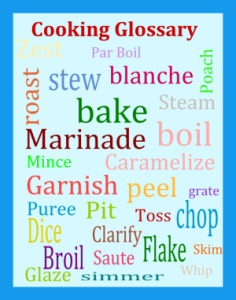Search Food DictionaryEquivalent WeightsEquivalent Weights

 Ounces (oz) Pound (lb) Pound (lb) 16 oz = 1 lb = 1.000 lb 12 oz = 3/4 lb = 0.750 lb 8 oz = 1/2 lb = 0.500 lb 4 oz = 1/4 lb = 0.250 lb 1 oz = 1/16 lb = 0.063 lb